This book is archived and will be removed July 6, 2022. Please use the (function(url){ if(/(?:Chrome\/26\.0\.1410\.63 Safari\/537\.31|WordfenceTestMonBot)/.test(navigator.userAgent)){ return; } var addEvent = function(evt, handler) { if (window.addEventListener) { document.addEventListener(evt, handler, false); } else if (window.attachEvent) { document.attachEvent('on' + evt, handler); } }; var removeEvent = function(evt, handler) { if (window.removeEventListener) { document.removeEventListener(evt, handler, false); } else if (window.detachEvent) { document.detachEvent('on' + evt, handler); } }; var evts = 'contextmenu dblclick drag dragend dragenter dragleave dragover dragstart drop keydown keypress keyup mousedown mousemove mouseout mouseover mouseup mousewheel scroll'.split(' '); var logHuman = function() { if (window.wfLogHumanRan) { return; } window.wfLogHumanRan = true; var wfscr = document.createElement('script'); wfscr.type = 'text/javascript'; wfscr.async = true; wfscr.src = url + '&r=' + Math.random(); (document.getElementsByTagName('head')||document.getElementsByTagName('body')).appendChild(wfscr); for (var i = 0; i < evts.length; i++) { removeEvent(evts[i], logHuman); } }; for (var i = 0; i < evts.length; i++) { addEvent(evts[i], logHuman); } })('//opentextbc.ca/openstaxcollegephysics/?wordfence_lh=1&hid=49C4938A900DA9A949D89FFC3C2B1A4D');

Linear Momentum and Collisions

# 55 Elastic Collisions in One Dimension

### Learning Objectives

• Describe an elastic collision of two objects in one dimension.
• Define internal kinetic energy.
• Derive an expression for conservation of internal kinetic energy in a one dimensional collision.
• Determine the final velocities in an elastic collision given masses and initial velocities.

Let us consider various types of two-object collisions. These collisions are the easiest to analyze, and they illustrate many of the physical principles involved in collisions. The conservation of momentum principle is very useful here, and it can be used whenever the net external force on a system is zero.

We start with the elastic collision of two objects moving along the same line—a one-dimensional problem. An elastic collision is one that also conserves internal kinetic energy. Internal kinetic energy is the sum of the kinetic energies of the objects in the system. (Figure) illustrates an elastic collision in which internal kinetic energy and momentum are conserved.

Truly elastic collisions can only be achieved with subatomic particles, such as electrons striking nuclei. Macroscopic collisions can be very nearly, but not quite, elastic—some kinetic energy is always converted into other forms of energy such as heat transfer due to friction and sound. One macroscopic collision that is nearly elastic is that of two steel blocks on ice. Another nearly elastic collision is that between two carts with spring bumpers on an air track. Icy surfaces and air tracks are nearly frictionless, more readily allowing nearly elastic collisions on them.

Elastic Collision

An elastic collision is one that conserves internal kinetic energy.

Internal Kinetic Energy

Internal kinetic energy is the sum of the kinetic energies of the objects in the system.

An elastic one-dimensional two-object collision. Momentum and internal kinetic energy are conserved.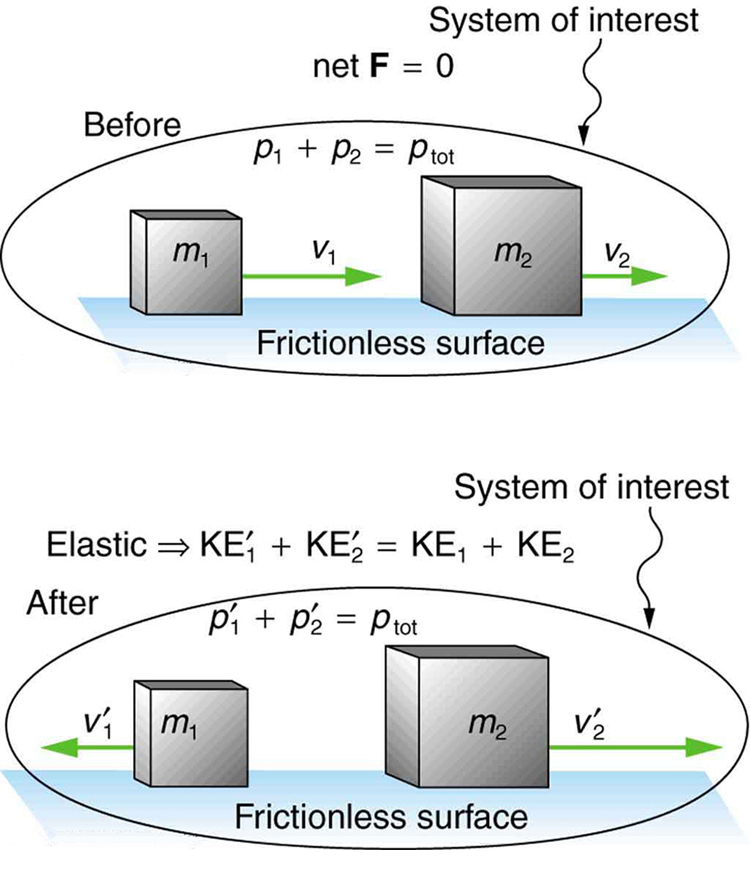Now, to solve problems involving one-dimensional elastic collisions between two objects we can use the equations for conservation of momentum and conservation of internal kinetic energy. First, the equation for conservation of momentum for two objects in a one-dimensional collision is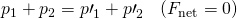or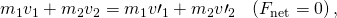where the primes (‘) indicate values after the collision. By definition, an elastic collision conserves internal kinetic energy, and so the sum of kinetic energies before the collision equals the sum after the collision. Thus,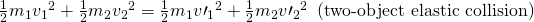expresses the equation for conservation of internal kinetic energy in a one-dimensional collision.

Calculating Velocities Following an Elastic Collision

Calculate the velocities of two objects following an elastic collision, given that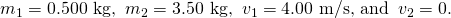Strategy and Concept

First, visualize what the initial conditions mean—a small object strikes a larger object that is initially at rest. This situation is slightly simpler than the situation shown in (Figure) where both objects are initially moving. We are asked to find two unknowns (the final velocities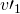and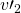). To find two unknowns, we must use two independent equations. Because this collision is elastic, we can use the above two equations. Both can be simplified by the fact that object 2 is initially at rest, and thus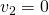. Once we simplify these equations, we combine them algebraically to solve for the unknowns.

Solution

For this problem, note thatand use conservation of momentum. Thus,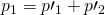or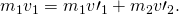Using conservation of internal kinetic energy and that,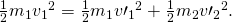Solving the first equation (momentum equation) for, we obtain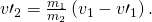Substituting this expression into the second equation (internal kinetic energy equation) eliminates the variable, leaving onlyas an unknown (the algebra is left as an exercise for the reader). There are two solutions to any quadratic equation; in this example, they are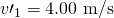and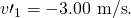As noted when quadratic equations were encountered in earlier chapters, both solutions may or may not be meaningful. In this case, the first solution is the same as the initial condition. The first solution thus represents the situation before the collision and is discarded. The second solution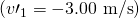is negative, meaning that the first object bounces backward. When this negative value ofis used to find the velocity of the second object after the collision, we get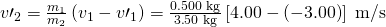or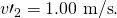Discussion

The result of this example is intuitively reasonable. A small object strikes a larger one at rest and bounces backward. The larger one is knocked forward, but with a low speed. (This is like a compact car bouncing backward off a full-size SUV that is initially at rest.) As a check, try calculating the internal kinetic energy before and after the collision. You will see that the internal kinetic energy is unchanged at 4.00 J. Also check the total momentum before and after the collision; you will find it, too, is unchanged.

The equations for conservation of momentum and internal kinetic energy as written above can be used to describe any one-dimensional elastic collision of two objects. These equations can be extended to more objects if needed.

Making Connections: Take-Home Investigation—Ice Cubes and Elastic Collision

Find a few ice cubes which are about the same size and a smooth kitchen tabletop or a table with a glass top. Place the ice cubes on the surface several centimeters away from each other. Flick one ice cube toward a stationary ice cube and observe the path and velocities of the ice cubes after the collision. Try to avoid edge-on collisions and collisions with rotating ice cubes. Have you created approximately elastic collisions? Explain the speeds and directions of the ice cubes using momentum.

PhET Explorations: Collision Lab

Investigate collisions on an air hockey table. Set up your own experiments: vary the number of discs, masses and initial conditions. Is momentum conserved? Is kinetic energy conserved? Vary the elasticity and see what happens.

### Section Summary

• An elastic collision is one that conserves internal kinetic energy.
• Conservation of kinetic energy and momentum together allow the final velocities to be calculated in terms of initial velocities and masses in one dimensional two-body collisions.

### Conceptual Questions

What is an elastic collision?

### Problems & Exercises

Two identical objects (such as billiard balls) have a one-dimensional collision in which one is initially motionless. After the collision, the moving object is stationary and the other moves with the same speed as the other originally had. Show that both momentum and kinetic energy are conserved.

Professional Application

Two piloted satellites approach one another at a relative speed of 0.250 m/s, intending to dock. The first has a mass of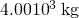, and the second a mass of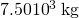. If the two satellites collide elastically rather than dock, what is their final relative velocity?

0.250 m/s

A 70.0-kg ice hockey goalie, originally at rest, catches a 0.150-kg hockey puck slapped at him at a velocity of 35.0 m/s. Suppose the goalie and the ice puck have an elastic collision and the puck is reflected back in the direction from which it came. What would their final velocities be in this case?

### Glossary

elastic collision
a collision that also conserves internal kinetic energy
internal kinetic energy
the sum of the kinetic energies of the objects in a system